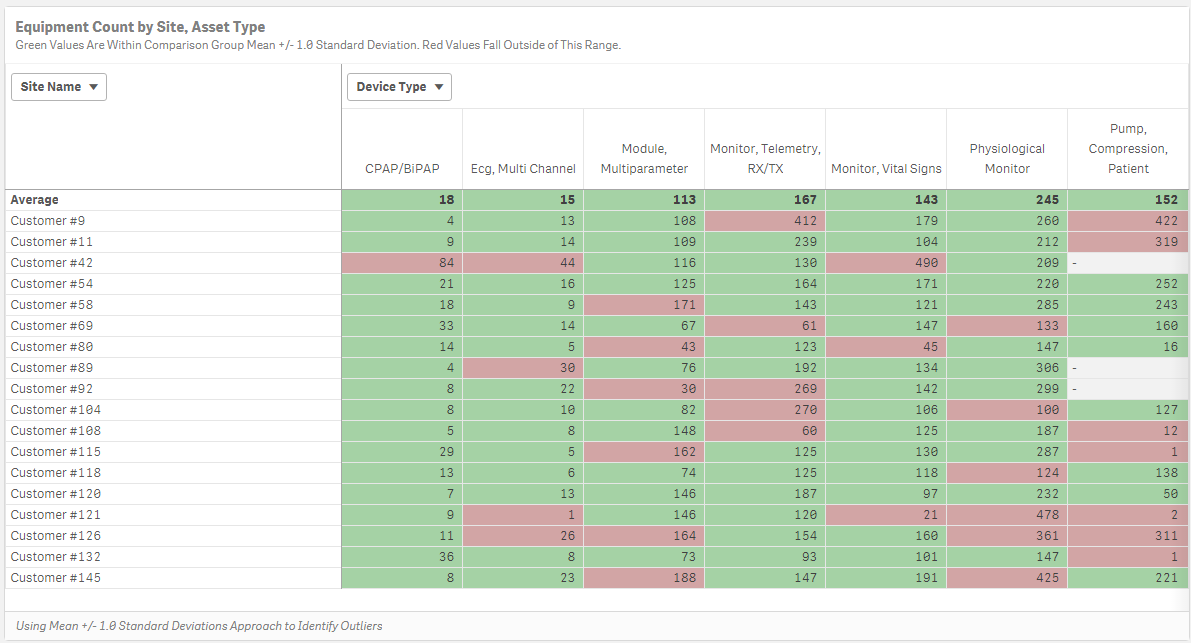New to Qlik Sense

Discussion board where members can get started with Qlik Sense.

New Contributor III

Set Analysis Help Needed

Hi!

I am working on creating a set analysis expression and need some help or pointers from the experts.

1. I calculate a number, using the following expression: =avg(aggr(sum(IB_COUNTER),[%ENCRYPTED_NAME], [%IB_DEVICE_TYPE]))

2. What I wan to do next is calculate a MAX((aggr(sum(IB_COUNTER),[%ENCRYPTED_NAME], [%IB_DEVICE_TYPE])), but only for the values that meet the following criteria:

=fabs(Sum(TOTAL<[%IB_DEVICE_TYPE]> IB_COUNTER) / COUNT(TOTAL<[%IB_DEVICE_TYPE]> DISTINCT [%ENCRYPTED_NAME]) - avg(aggr(sum(IB_COUNTER),[%ENCRYPTED_NAME], [%IB_DEVICE_TYPE]))) > \$(vOutliersThreshold) * Stdev(TOTAL<[%IB_DEVICE_TYPE]> Aggr(Sum(IB_COUNTER) ,[%IB_DEVICE_TYPE],[%ENCRYPTED_NAME]))

I think this could be done using indirect set analysis, but I am struggling with constructing the right expression.

GREATLY APPRECIATE ANY HELP!!

Tags (2)
1 Solution

Accepted SolutionsMVP

Re: Set Analysis Help Needed

Try this

If(Dimensionality() = 1, sum([Device Count]),

avg(aggr(if(fabs(Sum(TOTAL<DeviceType> [Device Count]) / COUNT(TOTAL<DeviceType> DISTINCT Customer) - avg(aggr(sum([Device Count]),Customer, DeviceType)))

<= 1*Stdev(TOTAL<DeviceType> Aggr(Sum([Device Count]) ,DeviceType,Customer)), sum([Device Count])),Customer, DeviceType)))10 RepliesMVP

Re: Set Analysis Help Needed

Would you be able to share a sample or some raw data and explain the expected output out of this sample?

New Contributor III

Re: Set Analysis Help Needed

Sunny,

Please see the picture below.I have color coded cells RED, when the value falls outside of Avg Value (first row) + 1 Std. Dev. These are outliers. Now, I would like to calculate the average value, for each Device Type, but only for cells that are green.MVP

Re: Set Analysis Help Needed

May be this

=Avg(Aggr(

If(fabs(Sum(TOTAL<[%IB_DEVICE_TYPE]> IB_COUNTER) / COUNT(TOTAL<[%IB_DEVICE_TYPE]> DISTINCT [%ENCRYPTED_NAME]) - avg(aggr(sum(IB_COUNTER),[%ENCRYPTED_NAME], [%IB_DEVICE_TYPE]))) > \$(vOutliersThreshold) * Stdev(TOTAL<[%IB_DEVICE_TYPE]> Aggr(Sum(IB_COUNTER) ,[%IB_DEVICE_TYPE],[%ENCRYPTED_NAME])),

Sum(IB_COUNTER), [%ENCRYPTED_NAME], [%IB_DEVICE_TYPE]))

New Contributor III

Re: Set Analysis Help Needed

Sunny, does not work.If you look at the image attached, value returned for the first Device Type (CPAP, BiPAP) should be 14 (average of all values in the CPAP/BiPAP column, excluding 84, the outlier).MVP

Re: Set Analysis Help Needed

What does the above give you?

Highlighted
New Contributor III

Re: Set Analysis Help Needed

Error. It does not return anythingMVP

Re: Set Analysis Help Needed

Would you be able to share a sample qvf file?

New Contributor III

Re: Set Analysis Help Needed

here it isMVP

Re: Set Analysis Help Needed

Try this

If(Dimensionality() = 1, sum([Device Count]),

avg(aggr(if(fabs(Sum(TOTAL<DeviceType> [Device Count]) / COUNT(TOTAL<DeviceType> DISTINCT Customer) - avg(aggr(sum([Device Count]),Customer, DeviceType)))

<= 1*Stdev(TOTAL<DeviceType> Aggr(Sum([Device Count]) ,DeviceType,Customer)), sum([Device Count])),Customer, DeviceType)))